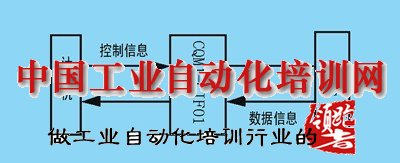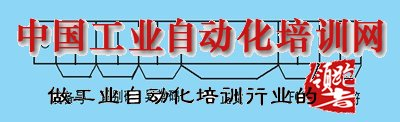| 網站導航 | 加入收藏010-63866300
18999183549

用VB實現PLC與計算機間的串行通信

1通訊原理與方法
上位機要能夠通過PLC監控下層設備的狀態，就要實現上位機與PLC間的通信，一般工業控制中都是采用RS232C實現。上位機首先向PLC發送查詢數據的指令(實際上是查詢PLC中端子的狀態和DM區的值等)PLC接收了上位的指令后，進行校驗(FCS校驗碼)，看其是否正確，如果正確，則向上位機傳送數據(包含首尾校驗字節)。否則，PLC拒絕向上位機傳送數據。上位接收到PLC傳送的數據，也要判斷正確與否，如果正確，則接收，否則，拒絕接收。2  PLC與計算機間的通信規約

(1)(2)為了充分利用計算機數據處理的強大功能，我們可以采用計算機有優先權的方式，在計算機上編寫程序來實現計算機與PLC的通信， 計算機向PLC發出命令發起通信，PLC自動返回響應。本文中采用VB來編寫計算機與PLC間的通訊程序。在VB中提供了通信控件應用通信控件(MSCOMM)，實現通過串行端口傳送和接受的功能。

*CommPort:

*PortOpen:

*Settings:

,d,s"
，其中bbbb為通信速率(波特率)p為通信檢查方式(奇偶校驗)  d     為數據位數，s為停止位數，其設定應與PLC的設定一致。

*Input:

*Output:

*InBufferCount:

*OutBufferCount:

*InputLen:

*InputMode:

*Rthreshold:

*CommEvent:

*OnComm

(1)

Mscomm.ComPort=2 '

Com2
Mscomm.Settings="9600, E, 7, 2" '

Mscomm.PortOpen=True '

(2)

Function FCS(ByVal InputStr As String) As String
Dim Slen, i, Xorresult As Integer
Dim Tempfes As String
Slen=Len(InputStr)      '

Xorresult = 0
For i = 1 To Slen
Xorresult = Xorresult Xor Asc(Mid\$(InputStr, i, 1))     '

Next i
Tempfes=Hex\$(Xorresult)     '

If Len(Tempfes)=1Then Tempfes =“0”+Tempfes
FCS = Tempfes
End Function
(3)

Function ReadData(ByVal InputStr As String, ByVal Num As Integer) As String
Dim OutputStr As String
Dim InString As String
Dim ReturnStr As String
Dim EndString As String
Dim FCSString As String
Dim ReturnFCSString As String)
MSComm.InBufferCount=0
OutputStr=InputStr+FCS(InputStr)+“*”   '

MSComm.Output=OutputStr+Chr\$(13)      '
PLC傳送命令幀
Do
DoEvents
Loop While MSComm.InBufferCount < 15
InString=MSComm.Input    '

'結束碼判斷
EndString = Mid\$(InString, Len(InString) -
Num- 5, 2)
If EndString = "13" Then
Exit Function
ElseIf EndString = "14" Then
Exit Function
ElseIf EndString = "15" Then
Exit Function
ElseIf EndString = "18" Then
Exit Function
ElseIf EndString = "A3" Then
Exit Function
ElseIf EndString = "A8" Then
Exit Function
End If

'響應幀校驗
EndString = Mid\$(InString, 1, Len(InString) - 4)
ReturnFCSString = Mid\$(InString,Len(InString) -     3, 2)
FCSString = FCS(EndString)
If FCSString <> ReturnFCSString Then
Exit Function
End If

ReturnStr = Mid\$(InString,
Len(InString) - Num - 3, Num)
End Function

聯系人：王老師                     郵箱：[email protected]
聯系電話：18999183549、0991-7799165、010-63866300
中國工業自動化培訓網址：www.icnoky.tw